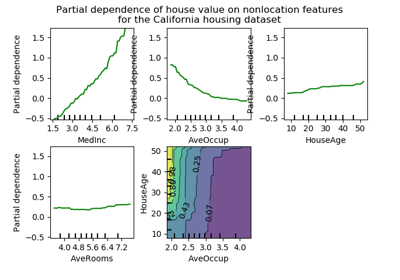/scikit-learn

# sklearn.ensemble.partial_dependence.plot_partial_dependence

`sklearn.ensemble.partial_dependence.plot_partial_dependence(gbrt, X, features, feature_names=None, label=None, n_cols=3, grid_resolution=100, percentiles=(0.05, 0.95), n_jobs=None, verbose=0, ax=None, line_kw=None, contour_kw=None, **fig_kw)` [source]

Partial dependence plots for `features`.

The `len(features)` plots are arranged in a grid with `n_cols` columns. Two-way partial dependence plots are plotted as contour plots.

Read more in the User Guide.

Parameters: `gbrt : BaseGradientBoosting` A fitted gradient boosting model. `X : array-like, shape=(n_samples, n_features)` The data on which `gbrt` was trained. `features : seq of ints, strings, or tuples of ints or strings` If seq[i] is an int or a tuple with one int value, a one-way PDP is created; if seq[i] is a tuple of two ints, a two-way PDP is created. If feature_names is specified and seq[i] is an int, seq[i] must be < len(feature_names). If seq[i] is a string, feature_names must be specified, and seq[i] must be in feature_names. `feature_names : seq of str` Name of each feature; feature_names[i] holds the name of the feature with index i. `label : object` The class label for which the PDPs should be computed. Only if gbrt is a multi-class model. Must be in `gbrt.classes_`. `n_cols : int` The number of columns in the grid plot (default: 3). `grid_resolution : int, default=100` The number of equally spaced points on the axes. `percentiles : (low, high), default=(0.05, 0.95)` The lower and upper percentile used to create the extreme values for the PDP axes. `n_jobs : int or None, optional (default=None)` `None` means 1 unless in a `joblib.parallel_backend` context. `-1` means using all processors. See Glossary for more details. `verbose : int` Verbose output during PD computations. Defaults to 0. `ax : Matplotlib axis object, default None` An axis object onto which the plots will be drawn. `line_kw : dict` Dict with keywords passed to the `matplotlib.pyplot.plot` call. For one-way partial dependence plots. `contour_kw : dict` Dict with keywords passed to the `matplotlib.pyplot.plot` call. For two-way partial dependence plots. `**fig_kw : dict` Dict with keywords passed to the figure() call. Note that all keywords not recognized above will be automatically included here. `fig : figure` The Matplotlib Figure object. `axs : seq of Axis objects` A seq of Axis objects, one for each subplot.

#### Examples

```>>> from sklearn.datasets import make_friedman1
>>> X, y = make_friedman1()
## Examples using `sklearn.ensemble.partial_dependence.plot_partial_dependence`